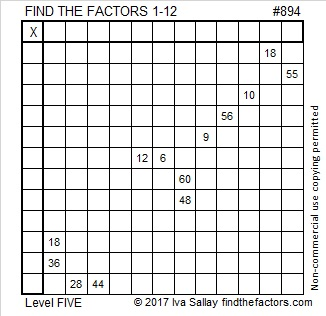# 894 and Level 5

894 is the hypotenuse of a Pythagorean triple: 306-840-894

894 is also palindrome 626 in BASE 12 because 6(144) + 2(12) + 6(1) = 894Print the puzzles or type the solution on this excel file: 12 factors 886-896

• 894 is a composite number.
• Prime factorization: 894 = 2 × 3 × 149
• The exponents in the prime factorization are 1, 1, and 1. Adding one to each and multiplying we get (1 + 1)(1 + 1)(1 + 1) = 2 × 2 × 2 = 8. Therefore 894 has exactly 8 factors.
• Factors of 894: 1, 2, 3, 6, 149, 298, 447, 894
• Factor pairs: 894 = 1 × 894, 2 × 447, 3 × 298, or 6 × 149
• 894 has no square factors that allow its square root to be simplified. √894 ≈ 29.89983This site uses Akismet to reduce spam. Learn how your comment data is processed.